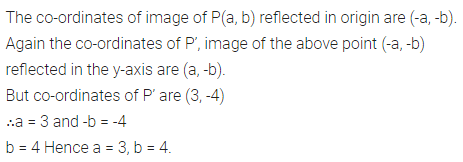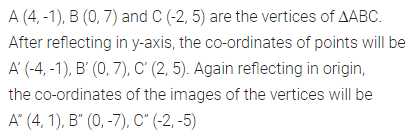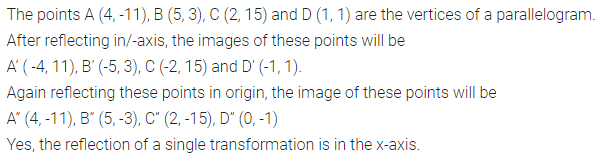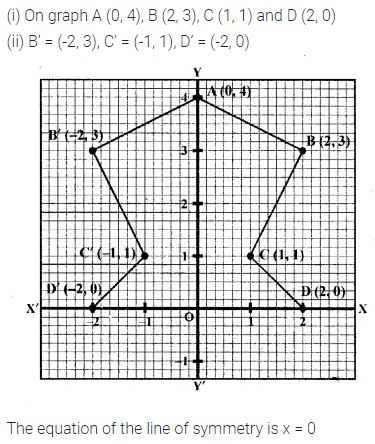# ML Aggarwal Class 10 Solutions for ICSE Maths Chapter 10 Reflection Chapter Test

## ML Aggarwal Class 10 Solutions for ICSE Maths Chapter 10 Reflection Chapter Test

ML Aggarwal Class 10 Solutions for ICSE Maths Chapter 10 Reflection Chapter Test

Question 1.
The point P (4, -7) on reflection in x-axis is mapped onto P’. Then P’ on reflection in the y-axis is mapped onto P”. Find the co-ordinates of P’ and P”. Write down a single transformation that maps P onto P”.
Solution:Question 2.
The point P (a, b) is first reflected in the origin and then reflected in the y-axis to P’. If P’ has co-ordinates (3, -4), evaluate a, b
Solution:Question 3.
A point P (a, b) becomes (-2, c) after reflection in the x-axis, and P becomes (d, 5) after reflection in the origin. Find the values of a, b, c and d.
Solution:Question 4.
A (4, -1), B (0, 7) and C (-2, 5) are the vertices of a triangle. ∆ABC is reflected in the y-axis and then reflected in the origin. Find the co-ordinates of the final images of the vertices.
Solution:Question 5.
The points A (4, -11), B (5, 3), C (2, 15), and D (1, 1) are the vertices of a parallelogram. If the parallelogram is reflected in the y-axis and then in the origin, find the co-ordinates of the final images. Check whether it remains a parallelogram. Write down a single transformation that brings the above change.
Solution:Question 6.
Use a graph paper for this question (take 2 cm = 1 unit on both x and y axes).
(i) Plot the following points:
A (0, 4), B (2, 3), C (1, 1) and D (2, 0).
(ii) Reflect points B, C, D on the y-axis and write down their coordinates. Name the images as B’, C’, D’ respectively.
(iii) Join points A, B, C, D, D’, C’, B’ and A in order, so as to form a closed figure. Write down the equation of line of symmetry of the figure formed. (2017)
Solution:Question 7.
The triangle OAB is reflected in the origin O to triangle OA’B’. A’ and B’ have coordinates (-3, -4) and (0, -5) respectively.
(i) Find the co-ordinates of A and B.
(ii) Draw a diagram to represent the given information.
(iii) What kind of figure is the quadrilateral ABA’B’?
(iv) Find the coordinates of A”, the reflection of A in the origin followed by reflection in the y-axis.
(v) Find the co-ordinates of B”, the reflection of B in the x-axis followed by reflection in the origin.
Solution:ML Aggarwal Class 10 Solutions for ICSE Maths